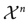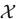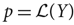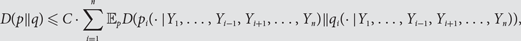Home

# Logarithmic Sobolev inequalities in discrete product spaces

## Abstract

The aim of this paper is to prove an inequality between relative entropy and the sum of average conditional relative entropies of the following form: for a fixed probability measure q on, (is a finite set), and any probability measureon, (*)$$D(p||q){\rm{\le}}C \cdot \sum\limits_{i = 1}^n {{\rm{\mathbb{E}}}_p D(p_i ( \cdot |Y_1 ,{\rm{ }}...,{\rm{ }}Y_{i - 1} ,{\rm{ }}Y_{i + 1} ,...,{\rm{ }}Y_n )||q_i ( \cdot |Y_1 ,{\rm{ }}...,{\rm{ }}Y_{i - 1} ,{\rm{ }}Y_{i + 1} ,{\rm{ }}...,{\rm{ }}Y_n )),}$$
where pi(· |y1, …, yi−1, yi+1, …, yn) and qi(· |x1, …, xi−1, xi+1, …, xn) denote the local specifications for p resp. q, that is, the conditional distributions of the ith coordinate, given the other coordinates. The constant C depends on (the local specifications of) q.

The inequality (*) ismeaningful in product spaces, in both the discrete and the continuous case, and can be used to prove a logarithmic Sobolev inequality for q, provided uniform logarithmic Sobolev inequalities are available for qi(· |x1, …, xi−1, xi+1, …, xn), for all fixed i and fixed (x1, …, xi−1, xi+1, …, xn). Inequality (*) directly implies that the Gibbs sampler associated with q is a contraction for relative entropy.

In this paper we derive inequality (*), and thereby a logarithmic Sobolev inequality, in discrete product spaces, by proving inequalities for an appropriate Wasserstein-like distance.

## Footnotes

Hide All

This work was supported by grant OTKA K 105840 of the Hungarian Academy of Sciences and by National Research, Development and Innovation Office NKFIH K 120706.

Hide All

# Logarithmic Sobolev inequalities in discrete product spaces

## Metrics

### Full text viewsFull text views reflects the number of PDF downloads, PDFs sent to Google Drive, Dropbox and Kindle and HTML full text views.

Total number of HTML views: 0
Total number of PDF views: 0 *Loading metrics...

### Abstract viewsAbstract views reflect the number of visits to the article landing page.

Total abstract views: 0 *Loading metrics...

* Views captured on Cambridge Core between <date>. This data will be updated every 24 hours.

Usage data cannot currently be displayed